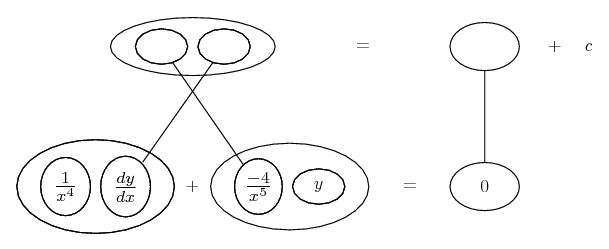# Thread: Basic Differential Equations

1. ## Basic Differential Equations

We have just had an introduction to differential equations, and I need a little help as I left a bit confused and am still fumbling around with two problems:

Suppose $\displaystyle Q=Ce^{-kt}$ and that $\displaystyle \frac {dQ} {dt} = -0.06Q$

What does this say about k and C?

At this point, I thought that $\displaystyle k = -.06$ and that $\displaystyle C$ could be any value at all, but there are apparently two different answers and I am not sure if what I had mentioned before is even correct.

The other one that I am having troubles with is:

$\displaystyle x\frac{dy} {dx} - 4y = 0$

I found that the general solution was $\displaystyle Cx^4$, but the problem asks for a second solution $\displaystyle y=Cx^n$ that might not be a general solution and which may have a different value of n than the one that was previously found. Could the answer be $\displaystyle y=0$ or is there an other answer that I am just not thinking of?

Thanks for the help everyone.

2.Originally Posted by SpudwadSuppose $\displaystyle Q=Ce^{-kt}$ and that $\displaystyle \frac {dQ} {dt} = -0.06Q$

What does this say about k and C?

At this point, I thought that $\displaystyle k = -.06$ and that $\displaystyle C$ could be any value at all, but there are apparently two different answers and I am not sure if what I had mentioned before is even correct.
Solve the differential equation. It is separable:

$\displaystyle \frac{dQ}{0.06Q} = -dt$

$\displaystyle \int \frac{dQ}{Q} = \int -0.06 dt$

$\displaystyle \ln Q = -0.06t + C_1$

$\displaystyle Q = e^{-0.06t + C_1}$

$\displaystyle Q = e^{C_1}e^{-0.06t}$

$\displaystyle Q = Ce^{-0.06t}$

Unless there is additional information, it seems that k = -0.06 and C could be any positive constant.

3. For the second, divide though by x, then multiply through by a suitable integrating factor... 1/x^4.

Edit: please excuse earlier typo. Also, just in case a picture helps...... where... is the product rule. Straight continuous lines differentiate downwards (integrate up) with respect to x.

So you now have an exact product-rule derivative on the left-had side, and you might be able to fill in the blanks. Then check by seeing, with or without using the pic, that differentiating gives you the bottom row.

As with the first problem, you haven't included any info that would enable you to then establish the value of c, the constant of integration. (But you perhaps aren't meant to.)

But how were you meant to choose 1/x^4 as the integrating factor? Basically, by making it e to the power of an anti-derivative of -4/x.

-4/x being what you have next to y after dividing through by x in the first place.

So how were you meant to know to divide through by x? See Integrating Factor
_________________________________________

Don't integrate - balloontegrate!

Balloon Calculus; standard integrals, derivatives and methods

Balloon Calculus Drawing with LaTeX and Asymptote!

#### Search Tags

basic, differential, equations